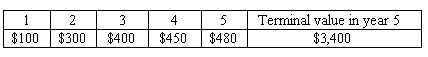### CFA Practice Question

There are 201 practice questions for this study session.

### CFA Practice Question

Consider the following information about a firm:

• Target debt ratio: 40%.
• Cost of equity: 0.20.
• Cost of debt: 9%.
• Tax rate: 30%.

The FCFF over the next five years and the terminal value of these cash flows at the end of year five are forecasted as shown below:What is the value of the firm?
A. \$2742.96
B. \$2813.84
C. \$2758.96
Explanation: WACC = 0.20 x 0.6 + 0.09 x (1 - 0.3) x 0.4 = 14.52%.

Value = 100/1.14521 + 300/1.14522 + 400/1.14523 + 450 / 1.14524 + 480/1.14525 + 3400/1.14525 = 2813.84.